# 使用注意力机制来做医学图像分割的解释和Pytorch实现

2020/08/14 09:08• 大多数医学图像都非常相似，因为它们是在标准化设置中拍摄的，这意味着在图像的方向、位置、像素范围等方面几乎没有变化。
• 通常在正样本像素(或体素)和负样本像素之间存在很大的不平衡，例如在尝试分割肿瘤时。

# 1. Attention UNet

UNet是用于分割的主要架构，目前在分割方面的大多数进展都使用这种架构作为骨干。在本文中，作者提出了一种将注意力机制应用于标准UNet的方法。

## 1.1. 提出了什么方法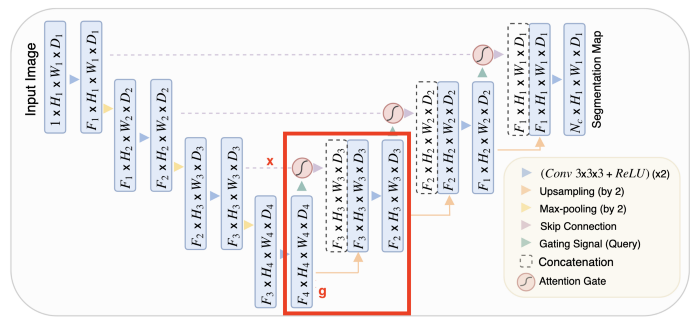attention UNet的框图，扩展路径block用红色框出upsample块非常简单，而ConvBlock只是由两个(convolution + batch norm + ReLU)块组成的序列。唯一需要解释的是注意力。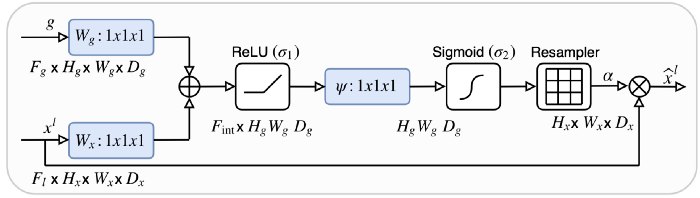• xg都被送入到1x1卷积中，将它们变为相同数量的通道数，而不改变大小
• 在上采样操作后(有相同的大小)，他们被累加并通过ReLU
• 通过另一个1x1的卷积和一个sigmoid，得到一个0到1的重要性分数，分配给特征图的每个部分
• 然后用这个注意力图乘以skip输入，产生这个注意力块的最终输出

## 1.3. 简短的实现

class AttentionBlock(nn.Module):    def __init__(self, in_channels_x, in_channels_g, int_channels):        super(AttentionBlock, self).__init__()        self.Wx = nn.Sequential(nn.Conv2d(in_channels_x, int_channels, kernel_size = 1),                                nn.BatchNorm2d(int_channels))        self.Wg = nn.Sequential(nn.Conv2d(in_channels_g, int_channels, kernel_size = 1),                                nn.BatchNorm2d(int_channels))        self.psi = nn.Sequential(nn.Conv2d(int_channels, 1, kernel_size = 1),                                 nn.BatchNorm2d(1),                                 nn.Sigmoid())        def forward(self, x, g):        # apply the Wx to the skip connection        x1 = self.Wx(x)        # after applying Wg to the input, upsample to the size of the skip connection        g1 = nn.functional.interpolate(self.Wg(g), x1.shape[2:], mode = 'bilinear', align_corners = False)        out = self.psi(nn.ReLU()(x1 + g1))        out = nn.Sigmoid()(out)        return out*xclass AttentionUpBlock(nn.Module):    def __init__(self, in_channels, out_channels):        super(AttentionUpBlock, self).__init__()        self.upsample = nn.ConvTranspose2d(in_channels, out_channels, kernel_size = 2, stride = 2)        self.attention = AttentionBlock(out_channels, in_channels, int(out_channels / 2))        self.conv_bn1 = ConvBatchNorm(in_channels+out_channels, out_channels)        self.conv_bn2 = ConvBatchNorm(out_channels, out_channels)        def forward(self, x, x_skip):        # note : x_skip is the skip connection and x is the input from the previous block        # apply the attention block to the skip connection, using x as context        x_attention = self.attention(x_skip, x)        # upsample x to have th same size as the attention map        x = nn.functional.interpolate(x, x_skip.shape[2:], mode = 'bilinear', align_corners = False)        # stack their channels to feed to both convolution blocks        x = torch.cat((x_attention, x), dim = 1)        x = self.conv_bn1(x)        return self.conv_bn2(x)

# 2. Multi-scale guided attention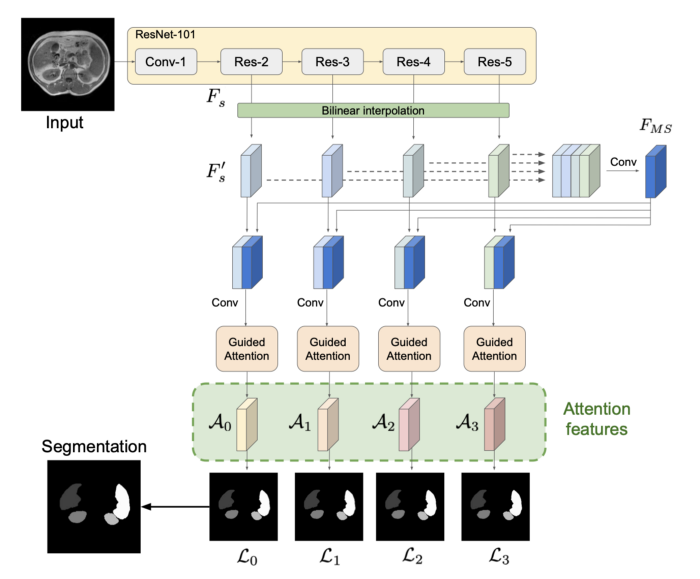## 2.1. 提出了什么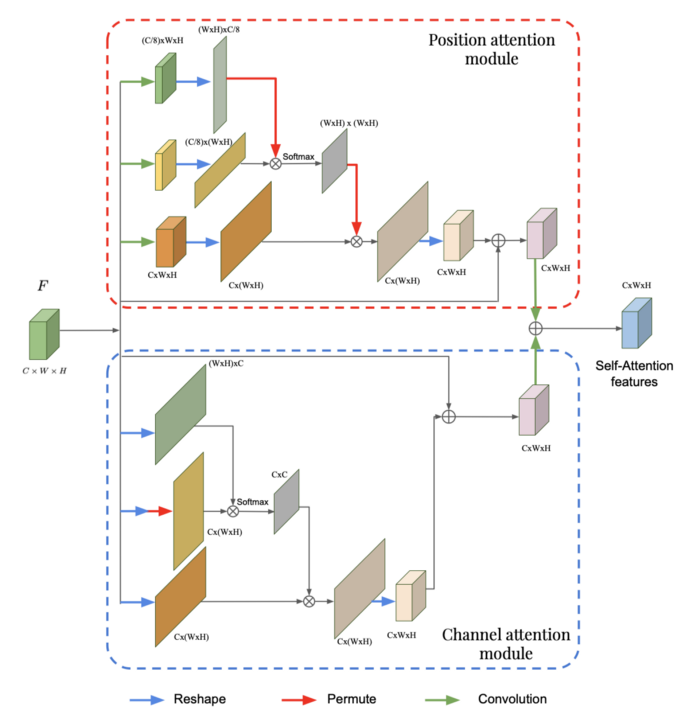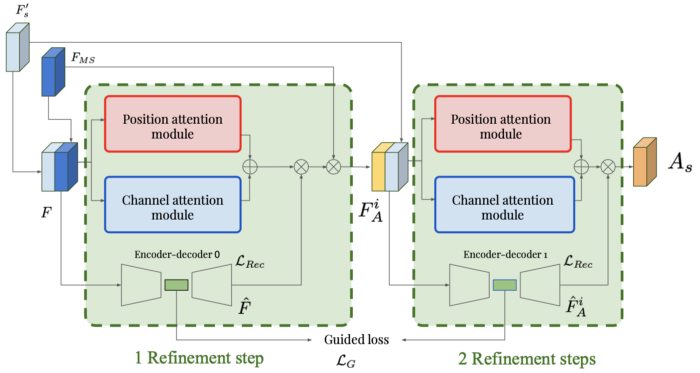• 标准重建损失，以确保自动编码器正确重建输入的特征图
• 引导损失，它试图最小化输入的两个后面的潜在表示之间的距离## 2.2. 为什么这样是有效的

autoencoder用来确保feature map的后续的表示在每一步之间都没有完全改变。由于潜空间是低维的，因此只提取关键信息。我们不希望将此信息从一个细化步骤更改为下一个细化步骤，我们只希望进行较小的调整。这些在潜在表示中不会被看到。

## 2.3. 简短的实现

class PositionAttentionModule(nn.Module):    def __init__(self, in_channels):        super(PositionAttentionModule, self).__init__()        self.first_branch_conv = nn.Conv2d(in_channels, int(in_channels/8), kernel_size = 1)        self.second_branch_conv = nn.Conv2d(in_channels, int(in_channels/8), kernel_size = 1)        self.third_branch_conv = nn.Conv2d(in_channels, in_channels, kernel_size = 1)        self.output_conv = nn.Conv2d(in_channels, in_channels, kernel_size = 1)        def forward(self, F):        # first branch        F1 = self.first_branch_conv(F)                  # (C/8, W, H)        F1 = F1.reshape((F1.size(0), F1.size(1), -1))   # (C/8, W*H)        F1 = torch.transpose(F1, -2, -1)                # (W*H, C/8)        # second branch        F2 = self.second_branch_conv(F)                 # (C/8, W, H)        F2 = F2.reshape((F2.size(0), F2.size(1), -1))   # (C/8, W*H)        F2 = nn.Softmax(dim = -1)(torch.matmul(F1, F2)) # (W*H, W*H)        # third branch        F3 = self.third_branch_conv(F)                  # (C, W, H)        F3 = F3.reshape((F3.size(0), F3.size(1), -1))   # (C, W*H)        F3 = torch.matmul(F3, F2)                       # (C, W*H)        F3 = F3.reshape(F.shape)                        # (C, W, H)        return self.output_conv(F3*F)class ChannelAttentionModule(nn.Module):    def __init__(self, in_channels):        super(ChannelAttentionModule, self).__init__()        self.output_conv = nn.Conv2d(in_channels, in_channels, kernel_size = 1)        def forward(self, F):        # first branch        F1 = F.reshape((F.size(0), F.size(1), -1))      # (C, W*H)        F1 = torch.transpose(F1, -2, -1)                # (W*H, C)        # second branch        F2 = F.reshape((F.size(0), F.size(1), -1))      # (C, W*H)        F2 = nn.Softmax(dim = -1)(torch.matmul(F2, F1)) # (C, C)        # third branch        F3 = F.reshape((F.size(0), F.size(1), -1))      # (C, W*H)        F3 = torch.matmul(F2, F3)                       # (C, W*H)        F3 = F3.reshape(F.shape)                        # (C, W, H)        return self.output_conv(F3*F)class GuidedAttentionModule(nn.Module):    def __init__(self, in_channels_F, in_channels_Fms):        super(GuidedAttentionModule, self).__init__()        in_channels = in_channels_F + in_channels_Fms        self.pam = PositionAttentionModule(in_channels)        self.cam = ChannelAttentionModule(in_channels)        self.encoder = nn.Sequential(nn.Conv2d(in_channels, 2*in_channels, kernel_size = 3),                                     nn.BatchNorm2d(2*in_channels),                                     nn.Conv2d(2*in_channels, 4*in_channels, kernel_size = 3),                                     nn.BatchNorm2d(4*in_channels),                                     nn.ReLU())        self.decoder = nn.Sequential(nn.ConvTranspose2d(4*in_channels, 2*in_channels, kernel_size = 3),                                     nn.BatchNorm2d(2*in_channels),                                     nn.ConvTranspose2d(2*in_channels, in_channels, kernel_size = 3),                                     nn.BatchNorm2d(in_channels),                                     nn.ReLU())        self.attention_map_conv = nn.Sequential(nn.Conv2d(in_channels, in_channels_Fms, kernel_size = 1),                                                nn.BatchNorm2d(in_channels_Fms),                                                nn.ReLU())            def forward(self, F, F_ms):        F = torch.cat((F, F_ms), dim = 1)         # concatenate the extracted feature map with the multi scale feature map        F_pcam = self.pam(F) + self.cam(F)        # sum the ouputs of the position and channel attention modules        F_latent = self.encoder(F)                # latent-space representation, used for the guided loss        F_reconstructed = self.decoder(F_latent)  # output of the autoencoder, used for the reconstruction loss        F_output = self.attention_map_conv(F_reconstructed * F_pcam)        F_output = F_output * F_ms        return F_output, F_reconstructed, F_latent

# 要点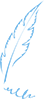END### 作者的其它热门文章

0
0 收藏

0 评论
0 收藏
0